# Key Signatures Quiz

To give you some practice with key signatures, here are some questions. Click the "check answer" button to see if you answered correctly.

1. What key signature is this?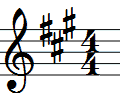Correct!

Incorrect.

2. What key signature is this?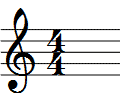Correct!

Incorrect.

3. What key signature is this?Correct!

Incorrect.

4. What key signature is this?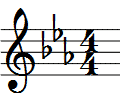Correct!

Incorrect.

5. What key signature is this?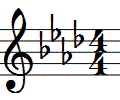Correct!

Incorrect.

6. What key signature is this?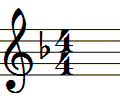Correct!

Incorrect.

7. What key signature is this?Correct!

Incorrect.

8. What key signature has 3 flats?

Correct!

Incorrect.

9. What key signature is this?Correct!

Incorrect.

10. What key signature is this?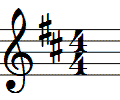Correct!

Incorrect.

11. What key signature is this?Correct!

Incorrect.

12. What key signature is this?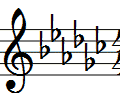Correct!

Incorrect.

13. How many sharps are in the key of F sharp?

Correct!

Incorrect.

14. What key signature is this?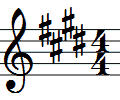Correct!

Incorrect.

15. What key signature is this?Correct!

Incorrect.

16. What key signature is this?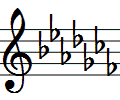Correct!

Incorrect.

17. How many sharps are in the key of F?

Correct!

Incorrect.

18. What key signature is this?Correct!

Incorrect.

19. What key signature has 4 flats?